| Next || Previous || Top |

## The Basic Interpreter

PHREEQC has an embedded Basic interpreter (David Gillespie, Synaptics, Inc., San Jose, Calif., written commun., 1997; distributed with the Linux operating system, Free Software Foundation, Inc.). Basic is a computer language with statements on numbered lines. The statements are much like the formulas entered in a spreadsheet cell, but Basic allows, in addition, the conditional statements and looping operations of a programming language. Variables can be defined at will, given a value, and used in subsequent lines. Variable names must start with a letter, which can be followed by any number of letters and numbers, and the variable's name must be different from the general and PHREEQC Basic functions. Names ending with a “\$” are for strings. Thus,

`10 A = 1.246 20 A\$ = 'A equals 1.246' `

is perfect.

In Basic you can use the operators “+”, “-”, “*”, “/”, and “=”, just as in written equations. A single variable is used on the left side of the equals sign. Expressions in parentheses, “(expression)”, are evaluated first, and then used in the more general expression. Exponentiation is done with the ^ sign: 2^2 = 4. The standard Basic and special PHREEQC Basic functions are listed in tables 7 and 8, respectively.

Basic programs are executed in line number order, regardless of the order used for writing the lines (but, for good programming, keep the number order intact). Basic variables, functions, and statements are case insensitive. Initially, a numeric variable is zero, and a string is empty, “”. The scope of variables is limited to the program unit where they are defined (RATES, USER_GRAPH, USER_PRINT, USER_PUNCH, or CALCULATE_VALUES data block). Numeric data can be transferred between program units with the functions PUT and GET (see table 8). However, in multithreaded and multiprocessor applications (for instance, PHAST, Parkhurst and others, 2010), PUT and GET may not work correctly.

Basic in PHREEQC is quite powerful, and it could be used for other purposes than manipulating variables in PHREEQC. For example, the following input file (illustrated in figure 3) plots the sine function from 0 to 360 degrees:

`SOLUTION 1 REACTION; H2O 0; 0 in 21 USER_GRAPH -axis_titles 'ANGLE, IN DEGREES' 'SINE(ANGLE)' -axis_scale x_axis 0 360 90 10 pi = 2 * arctan(1e20) 20 i = pi * (step_no - 1) / 10 30 graph_x i * 180 / pi 40 graph_y sin(i) END`

Originally, the Basic interpreter was a unique feature of PHREEQC version 2, aimed at calculating rates for kinetic geochemical processes. Rate expressions for kinetic reactions can have various forms, and they tend to be redefined or updated frequently as more data become available. In a PHREEQC input file, the rates can be adapted easily by the user as necessary. Because rate expressions often depend on conditions (for example, the rate expression may be different for mineral dissolution and precipitation), the conditional “if” statement of Basic can be necessary. Special Basic functions (table 8) have been written to retrieve geochemical quantities that frequently are used in kinetic rate expressions, such as molalities, activities, saturation indices, and moles of reactants.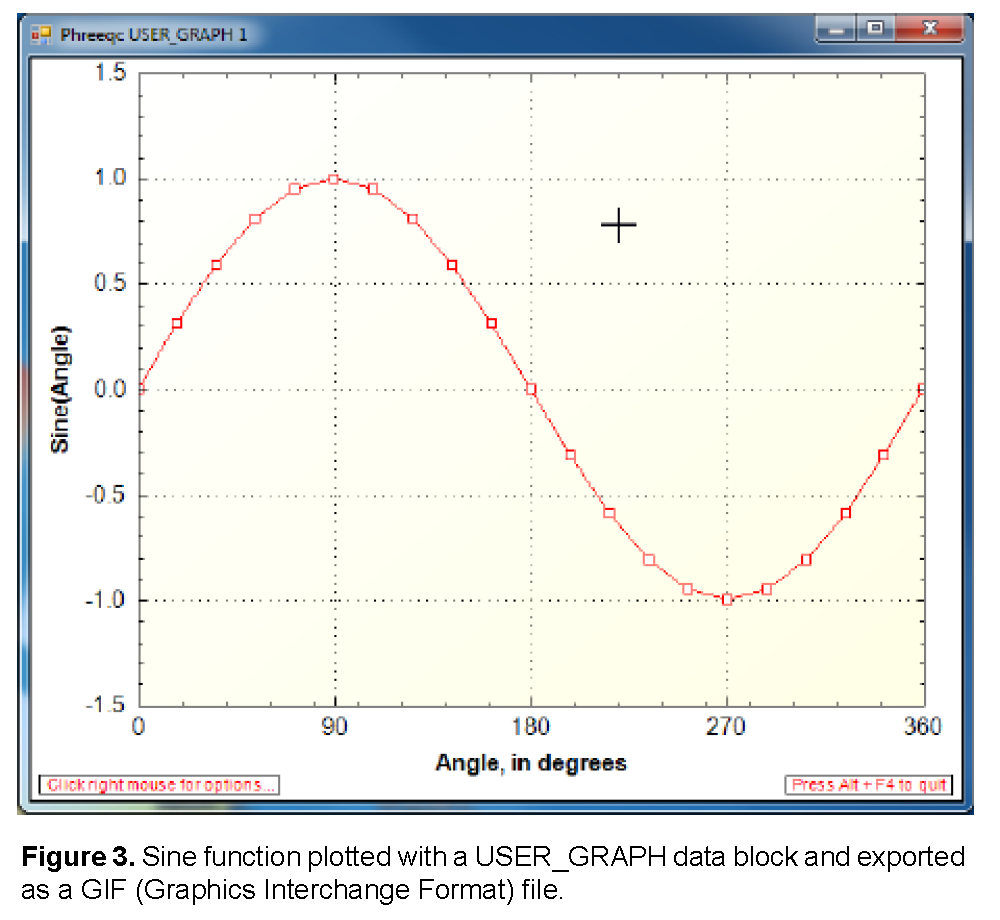PHREEQC calculations generate a large number of geochemical quantities, possibly distributed in space and time as well. Rather than storing or writing all of these quantities for a run, small Basic programs in the data blocks USER_GRAPH, USER_PRINT and USER_PUNCH can be used to print selected items or to calculate and graph specific numbers such as sums of species or concentrations in milligrams per liter. Also, the implementation of isotopes as individual chemical components relies heavily on Basic programs in the CALCULATE_VALUES data block, where Basic is used to calculate specific isotopic ratios, such as of carbon-13 in various bicarbonate species. Functions defined in CALCULATE_VALUES data blocks can be used in any Basic program within PHREEQC.

Table 7. Standard Basic statements and functions.

Basic Statements and Functions

Explanation

+, -, *, /

string1 + string2

String concatenation.

a ^ b

Exponentiation,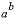.

<, >, <=, >=, <>, =,

AND, OR, XOR, NOT

Relational and Boolean operators.

ABS( a )

Absolute value.

ARCTAN( a )

Arctangent function.

ASC( character )

ASCII value for character.

CHR\$( number )

Convert ASCII number to character.

CEIL(a)

Smallest integer not less than a.

COS( a )

Cosine function.

DATA list

List of data.

DIM a ( n )

Define a dimensioned variable.

END

Ends the program

EOL\$

End of line string that is appropriate for the operating system.

ERASE v

Revert the Basic variable to an undimensioned variable so that it can be used as a scalar or dimensioned with another DIM statement. Applies only to variables that have been dimensioned with a DIM statement.

EXP( a )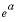.

FLOOR(a)

Largest integer not greater than a.

FOR i = n TO m STEP k

NEXT i

“For” loop.

GOSUB line

Go to subroutine at line number.

GOTO line

Go to line number.

IF ( expr ) THEN statement ELSE statement

If, then, else statement (on one line; a “\” may be used to concatenate lines).

INSTR( a\$ . b\$ )

The character position of the beginning of string b\$ in a\$ ; 0 if not found.

LEN( string )

Number of characters in string.

LOG( a )

Natural logarithm.

LOG10( a )

Base 10 logarithm.

LTRIM( a \$)

Trims white space from the beginning of string a\$ .

MID\$( string, n )

MID\$( string, n, m )

Extract characters from position n to end of string.

Extract m characters from string starting at position n .

a MOD b

Returns remainder of a / b .

ON expr GOTO line1 , line2 , ...

ON expr GOSUB line1 , line2 , ...

If the value of the expression, rounded to an integer, is N , go to the N th line number in the list. If N is less than one or greater than the number of line numbers listed, execution continues at the next statement after the ON statement.

Pads a\$ with spaces to a total of i characters; returns a copy of a\$ if the length of a\$ is more than i characters.

REM

At beginning of line, line is a remark with no effect on the calculations.

RESTORE line

Set pointer to DATA statement at line for subsequent READ.

RETURN

Return from subroutine.

RTRIM( a\$ )

Trims white space from the end of string a\$ .

SGN( a )

Sign of a , +1 or -1.

SIN( a )

Sine function.

SQR( a )

a 2 .

SQRT( a )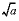.

STR\$( a )

Convert number to a string. The string may or may not have an exponential format depending on the value of a.

STR_E\$(a,w,d)

Converts number (a) to a string with exponential format (for example, “-1.234e-002”). The width of the string is w (or longer if more characters are needed), and the number of decimal places is d.

STR_F\$(a,w,d)

Converts number (a) to a string that has no exponent (for example, “-0.01234”). The width of the string is w (or longer if more characters are needed), and the number of decimal places is d.

TAN( a )

Tangent function.

TRIM( a\$ )

Trims white space from the beginning and end of string a\$ .

VAL( string )

Convert string to number.

WHILE ( expression )

WEND

“While” loop.

Table 8. Special Basic statements and functions for PHREEQC.

Special PHREEQC
Statement or Function

Explanation

ACT("HCO3-")

Activity of an aqueous, exchange, or surface species.

ALK

Alkalinity of solution, equivalents per kilogram water.

APHI

The A(phi) parameter of the Pitzer formulation of aqueous thermodynamics at the current solution conditions.

CALC_VALUE("R(D)_OH-")

Value calculated by Basic function (here, “R(D)_OH-”) defined in CALCULATE_VALUES data block.

CELL_NO

Cell number in TRANSPORT or ADVECTION calculations; otherwise solution or mix number.

CHANGE_POR(0.21, cell_no)

Modifies the porosity in a cell, used only in multicomponent diffusion calculations (keyword TRANSPORT ). Here, porosity of cell cell_no is set to 0.21.

CHANGE_SURF("Hfo", 0.2, "Sorbedhfo", 0, cell_no)

Changes the diffusion coefficient of (part of) a surface ( SURFACE ), and renames the surface (if names are different). This function is for modeling transport, deposition, and remobilization of colloids. It is used in conjunction with multicomponent diffusion in a TRANSPORT data block. Here: take a fraction 0.2 of “Hfo” and rename it “Sorbedhfo” with a diffusion coefficient of 0, in cell cell_no. The diffusion coefficient of zero means that “Sorbedhfo” is not transported.

CHARGE_BALANCE

Charge balance of a solution, equivalents.

CURRENT_A

The electrical current through the column, in amperes, when simulating electro-migration in TRANSPORT .

DESCRIPTION

Description associated with current solution or current mixture.

DIFF_C(“CO3-2”)

Diffusion coefficient at 25 C for the specified aqueous species.

DH_A

Debye-Hückel A parameter in the activity coefficient equation, (mol/kg) -0.5 .

DH_Av

Debye-Hückel limiting slope of specific volume vs. ionic strength, (cm 3 /mol) (mol/kg) -0.5 .

DH_B

Debye-Hückel B parameter in the activity coefficient equation, angstrom -1 (mol/kg) -0.5 .

DIST

Distance to midpoint of cell in TRANSPORT calculations, cell number in ADVECTION calculations, “-99” in all other calculations.

EDL("As", "Hfo")

Moles of element in the diffuse layer of a surface. The number of moles does not include the specifically sorbed species. The surface name should be used, not a surface site name (that is, no underscore). The first argument can have several special values, which return information for the surface: “charge”, surface charge, in equivalents; “sigma”, surface charge density, coulombs per square meter; “psi”, potential, Volts; “water”, mass of water in the diffuse layer, kg.

For CD-MUSIC surfaces, charge, sigma and psi can be requested for the 0, 1 and 2 planes:
EDL("Charge", "Goe") # Charge (eq) at the zero-plane of Goe (Goethite)
EDL("Charge1", "Goe") # Charge (eq) at plane 1 of Goe
EDL("Charge2", "Goe") # Charge (eq) at plane 2 of Goe
and similar for “sigma” and “psi”.

EDL_SPECIES(surf\$, count, name\$, moles, area, thickness)

Returns the total number of moles of species in the diffuse layer. The The arguments to the function are as follows: surf\$ is the name of a surface, such as "Hfo", excluding the site type (such as "_s"); count is the number of species in the diffuse layer; name\$ is an array of size count that contains the names of aqueous species in the diffuse layer of surface surf\$; moles is an array of size count that contains the number of moles of each aqueous species in the diffuse layer of surface surf\$; area is the area of the surface in m^2; thickness is the thickness of the diffuse layer in m. The function applies when -donnan or -diffuse_layer is defined in SURFACE calculations.

EOL\$

End of line character, which is equivalent to “\n” in the C programming language.

EPS_R

Relative dielectric constant.

EQUI("Calcite")

Moles of a phase in the equilibrium-phase assemblage.

EQUI_DELTA("Calcite")

Moles of a phase in the equilibrium-phase assemblage that reacted during the current calculation.

EQUIV_FRAC("(Hfo_w)2Al+", eq, x\$)

Equivalent fraction of an exchange or surface species relative to the total number of equivalents of exchange or surface sites. The second argument returns the number of sites per mole of species. The third argument returns the site name (“Hfo_w” in the example). If an exchange or surface species is not found with the given name, the function returns zero; the second argument is zero, and the third argument is an empty string.

EXISTS( i1 [ , i2, ... ])

Determines if a value has been stored with a PUT statement for the list of one or more subscripts.The function equals 1 if a value has been stored and 0 if no value has been stored. Values are stored in global storage with PUT and are accessible by any Basic program. See description of PUT for more details.

GAMMA("H+")

Activity coefficient of a species.

GAS("CO2(g)")

Moles of a gas component in the gas phase.

GAS_P

Pressure of the GAS_PHASE (atm), either specified for a fixed-pressure gas phase, or calculated for a fixed-volume gas phase. Related functions are PR_P and PRESSURE.

GAS_VM

Molar volume (L/mol, liter per mole) of the GAS_PHASE (calculated with Peng-Robinson).

GET( i1 [ , i2, ... ])

Retrieves the value that is identified by the list of one or more subscripts.Value is zero if PUT has not been used to store a value for the set of subscripts. Values stored in global storage with PUT are accessible by any Basic program. See description of PUT for more details.

GET_POR(10)

Porosity in a cell (here, cell 10), used in conjunction with Basic function CHANGE_POR in multicomponent diffusion.

GFW("CaCO3")

Returns the gram formula weight of the specified formula.

GRAPH_X tot("Ca") * 40.08e3

Used in USER_GRAPH data block to define the X values for points. Here, Ca in mg/L is the X value for points of the chart. See the description of the USER_GRAPH keyword for more details.

GRAPH_Y tot("F") * 19e3

Used in USER_GRAPH data block to define the Y values for points plotted on the primary Y axis. Here, F in mg/L is the Y value for points. See the description of the USER_GRAPH keyword for more details.

GRAPH_SY-la("H+")

Used in USER_GRAPH data block to define the Y values for points plotted on the secondary Y axis. Here, pH is the Y value for points plotted on the secondary Y axis. See the description of the USER_GRAPH keyword for more details.

ISO("[18O]"), ISO("R(D)_H3O+")

Isotopic composition in the input units (for example, permil) for an isotope (here, [18O]) or an isotope ratio defined in ISOTOPE_RATIOS (here, “R(D)_H3O+”).

ISO_UNIT("[18O]"), ISO_UNIT("R(D)_H3O+")

String value for the input units (for example, “permil”) for an isotope or an isotope ratio defined in ISOTOPE_RATIOS .

KAPPA

Compressibility of pure water at current pressure and temperature.

KIN("CH2O")

Moles of a kinetic reactant.

KIN_DELTA("CH2O")

Moles of a kinetic reactant that reacted during the current calculation.

KIN_TIME

Time interval in seconds of the last kinetic integration. KIN_DELTA("CH2O")/KIN_TIME will give the average rate over the time interval for reaction CH2O.

KINETICS_FORMULA\$("Albite", count, elt\$, coef)

KINETICS_FORMULA\$ returns a string that contains the first argument of the argument list if the kinetic reaction defined by the first argument is found, or a blank string if not. In addition, values are returned for count, elt\$, and coef. Count is the dimension of the elt\$ and coef arrays. Elt\$ is a character array with the name of each element in the chemical formula defined for the kinetic reaction. Coef is a numeric array containing the number of atoms of each element in the kinetic reaction formula, in the order defined by elt\$, which is alphabetical by element.

LA("HCO3-")

Log10 of activity of an aqueous, exchange, or surface species.

LG("H+")

Log10 of the activity coefficient for an aqueous species.

LIST_S_S("Carbonate_s_s", count, comp\$, moles)

Returns the sum of the moles of components in a solid solution and the composition of the solid solution. The first argument is an input value specifying the name of the solid solution. Count is an output variable containing the number of components in the solid solution. Comp\$ is an output character array containing the names of each component in the solid solution. Moles is an output numeric array containing the number of moles of each component, in the order defined by Comp\$. Arrays are in sort order by number of moles.

LK_NAMED("Log_alpha_D_OH-/H2O(l)")

The value calculated by a named expression defined in the MIX_EQUILIBRIUM_PHASES data block.

LK_PHASE("Calcite")

Log10 of the equilibrium constant for a phase defined in the PHASES data block.

LK_SPECIES("HCO3-")

Log10 of the equilibrium constant for an aqueous, exchange, or surface species.

LM("HCO3-")

Log10 of molality of an aqueous, exchange, or surface species.

M

Current moles of the kinetic reactant for which the rate is being calculated (see KINETICS ).

M0

Initial moles of the kinetic reactant for which the rate is being calculated (see KINETICS ).

MISC1("Ca(x)Sr(1-x)SO4")

Mole fraction of component 2 at the beginning of the miscibility gap, returns 1.0 if there is no miscibility gap (see SOLID_SOLUTIONS ).

MISC2("Ca(x)Sr(1-x)SO4")

Mole fraction of component 2 at the end of the miscibility gap, returns 1.0 if there is no miscibility gap (see SOLID_SOLUTIONS ).

MOL("HCO3-")

Molality of an aqueous, exchange, or surface species.

MU

Ionic strength of the solution.

OSMOTIC

Osmotic coefficient if using the Pitzer or SIT aqueous model, otherwise 0.0, unitless.

PARM( i )

The ith item in the parameter array defined in KINETICS data block.

PERCENT_ERROR

Percent charge-balance error [100(cations-|anions|)/(cations + |anions|)], unitless.

PHASE_FORMULA \$ ("Dolomite ")

With a single argument, PHASE_FORMULA\$ returns a string that contains the chemical formula for the phase; in this example, “CaMg(CO3)2”.

PHASE_FORMULA \$ ("Dolomite", count , elt\$ , coef)

With four arguments, PHASE_FORMULA\$ returns a string that contains the chemical formula for the phase, and, in addition, returns values for count , elt\$ , coef. Count is the dimension of the elt\$ and coef arrays. Elt\$ is a character array with the name of each element in the chemical formula for the phase. Coef is a numeric array containing the number of atoms of each element in the phase formula, in the order defined by elt\$, which is alphabetical by element.

PHASE_VM("Calcite")

Returns the molar volume for a mineral (cm 3 /mol). The molar volume is defined for the mineral in PHASES with the -vm option. Use the Basic function GAS_VM for gas components.

PLOT_XY tot("Ca") * 40.08e3, tot("F") * 19e3, color = Blue, symbol = Circle, symbol_size = 6, y-axis = 1, line_width = 0

Used in USER_GRAPH data block to define the points to chart; here, Ca in mg/L is the X value for points, F in mg/L is the Y value for points, the symbols are blue circles, the points are plotted relative to the Y axis, and no line connects the points. See the description of the USER_GRAPH keyword for more details.

POT_V

Potential in a cell, in Volts.

PRINT

Write to output file.

PR_P("CO2(g)")

Pressure (atm) of a gas component in a Peng-Robinson GAS_PHASE.

PR_PHI("CO2(g)")

Fugacity coefficient of a gas component in a Peng-Robinson GAS_PHASE.

PRESSURE

Current pressure applied to the solution (atm). PRESSURE is a specified value except for fixed-volume GAS_PHASE calculations.

PUNCH

Write to selected-output file.

PUT( x , i1 [ , i2, ... ])

Saves value of x in global storage that is identified by a sequence of one or more subscripts. Value of x can be retrieved with GET( i1, [ , i2, ... ]) and a set of subscripts can be tested to determine if a value has been stored with EXISTS( i1 [ , i2, ... ]). PUT may be used in CALCULATE_VALUES , RATES , USER_GRAPH , USER_PRINT , or USER_PUNCH Basic programs to store a value. The value may be retrieved by any of these Basic programs. The value persists until overwritten by using a PUT statement with the same set of subscripts, or until the end of the run. For a KINETICS data block, the Basic programs for the rate expressions are evaluated in the order in which they are defined in the input file. Use of PUT and GET in parallel processing environments may be unreliable.

QBRN

The Born parameter for calculating the temperature dependence of the specific volume of an aqueous species at infinite dilution. This is the pressure derivative of the relative dielectric constant of water multiplied by 41.84 bar cm 3 /cal (bar cubic centimeter per calorie):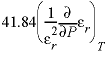, cm 3 /mol

RHO

Density of solution, kilograms per liter.

RHO_0

Density of pure water at the current temperature and pressure, kilograms per liter.

RXN

Moles of reaction as defined in -steps in REACTION data block for a batch-reaction calculation; otherwise zero.

SAVE

Moles of kinetic reactant for a time step in a rates function or the value returned from a CALCULATE_VALUES function.

SC

Specific conductance, microsiemens per centimeter.

SI("Calcite")

Saturation index of a phase,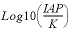log 10 of the ion activity product divided by equilibrium constant. For gases, this value is equal to log10(fugacity). For ideal gases, fugacity equals partial pressure. For Peng-Robinson gases, the Basic functions PR_P and PR_PHI can be used to obtain the gas partial pressure and the fugacity coefficient.

SIM_NO

Simulation number, equals one more than the number of END statements before current simulation.

SIM_TIME

Time from the beginning of a kinetic batch-reaction or transport calculation, in seconds.

SOLN_VOL

Volume of the solution, in liters.

SPECIES_FORMULA\$("AlOH4-", count, elt\$, coef)

SPECIES_FORMULA\$ returns a string that contains the type of the species--“aq”, “ex”, “surf”, or “none” if the species name is not found. In addition, values are returned for count, elt\$, and coef. Count is the dimension of the elt\$ and coef arrays. Elt\$ is a character array with the name of each element in the chemical formula for the species plus an entry for “charge” (the charge number of the species). Coef is a numeric array containing the number of atoms of each element in the species formula, in the order defined by elt\$, which is alphabetical by element.

SR("Calcite")

Saturation ratio of a phase,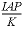, ion activity product divided by equilibrium constant. For gases, SR returns the fugacity of the gas (P*phi/1 atm).

STEP_NO

Step number in batch-reaction calculations, or shift number in ADVECTION and TRANSPORT calculations.

SUM_GAS("template", "element")

Sums number of moles of the element in gases that match the template. The template selects a set of gases. For example, a template of “{C,[13C],[14C]}{O,[18O]}2” selects all the isotopic variants of CO2(g). Multiple elements at a stoichiometric position are separated by commas within braces; an asterisk (*) in the template matches any element. The number of moles of “element” is calculated by summing the stoichiometric coefficient of the element times the moles of the gas for all selected gases.

SUM_SPECIES("template", "element")

Sums number of moles of the element in aqueous, exchange, and surface species that match the template. The template selects a set of species. For example, a template of “*HCO3*” selects all bicarbonate species. Multiple elements at a stoichiometric position are separated by commas within braces; an asterisk (*) in the template matches any element. The number of moles of “element” is calculated by summing the stoichiometric coefficient of the element times the moles of the species for all selected species.

SUM_S_S("s_s_name", "element")

Sums number of moles of the element in the specified solid solution.

SURF("element", "surface")

Number of moles of the element sorbed on the surface. The second argument should be the surface name, not the surface-site name (that is, no underscore). A redox state may be specified; for example, “As” or “As(5)” is permitted.

SYS("element")

With a single argument, SYS calculates the number of moles of the element in all phases (solution, equilibrium phases, surfaces, exchangers, solid solutions, and gas phase) in the reaction calculation.

SYS("element", count , name\$ , type\$ , moles )

With five arguments, SYS returns the number of moles of the element in all phases in the reaction calculation (solution, equilibrium phases, surfaces, exchangers, solid solutions, and gas phase), and, in addition, returns values for count_species , name\$ , type\$ , moles. Count is the dimension of the name\$ , type\$ , and moles arrays. Name\$ is a character array with the name of each species that contains the element. Type\$ , is a character array with the type of the phase of each species: “aq”, “equi”, “surf”, “ex”, “s_s”, “gas”, or “diff”; where aq is aqueous, equi is equilibrium phase, surf is surface, ex is exchange, s_s is solid solution, gas is gas phase, and diff is surface diffuse layer. Moles is the number of moles of the element in the species (stoichiometry of element times moles of species). The sum of all items in the moles array is equal to the return value of the SYS function.

The five-argument form of SYS accepts the following arguments in place of “element”:

elements ” returns the total number of moles of elements solution, exchangers, and surfaces in the calculation, other than H and O. Count is number of elements, valence states, exchangers, and surfaces. Name\$ contains the element name. Type\$ contains the type for each array item: “dis” for dissolved, “ex” for exchange, and “surf” for surface. Moles contains the number of moles of the element in each type of phase (stoichiometry of element times moles of species).

phases ” returns the maximum saturation index of all pure phases appropriate for the calculation. Count is number of pure phases. Name\$ contains the phase names as defined in the PHASES data block. Type\$ is “phase”. Moles contains the saturation index for the phases.

aq ” returns the sum of moles of all aqueous species in the calculation. Count is number of aqueous species. Name\$ contains the aqueous species names. Type\$ is “aq”. Moles contains the moles of species.

“equi” returns the sum of moles of all equilibrium phases in the calculation. Count is number of equilibrium phases. Name\$ contains the equilibrium phase names. Type\$ is “equi”. Moles contains the moles of each equilibrium phase.

ex ” returns the sum of moles of all exchange species in the calculation. Count is number of exchange species. Name\$ contains the exchange species names. Type\$ is “ex”. Moles contains the moles of species.

“kin” returns the sum of moles of all kinetic reactants in the calculation. Count is number of kinetic reactants. Name\$ contains the kinetic reactant names. Type\$ is “kin”. Moles contains the moles of each kinetic reactant. The chemical formula used in the kinetic reaction can be determined by using a reaction name from Name\$ as the first argument of the KINETICS_FORMULA\$ Basic function.

surf ” returns the sum of moles of all surface species in the calculation. Count is number of surface species. Name\$ contains the surface species names. Type\$ is “surf”. Moles contains the moles of species.

s_s ” returns sum of moles of all solid-solution components in the calculation. Count is number of solid-solution components. Name\$ contains the names of the solid-solution components. Type\$ is “s_s”. Moles contains the moles of components.

gas ” returns sum of moles of all gas components in the calculation. Count is number of gas components. Name\$ contains names of the gas components. Type\$ is “gas”. Moles contains the moles of gas components

S_S("Magnesite")

Current moles of a solid-solution component.

TC

Temperature in Celsius.

TK

Temperature in Kelvin.

TIME

Time interval for which moles of reaction are calculated in rate programs, automatically set in the time-step algorithm of the numerical integration method, in seconds.

TITLE

Returns string value of the last TITLE keyword definition (with tabs removed).

TOT("Fe(2)")

Total molality of element or element redox state. TOT(“water”) is total mass of water, in kilograms.

TOTAL_TIME

Cumulative time (seconds) including all advective (for which -time_step is defined) and advective-dispersive transport simulations from the beginning of the run or from last -initial_time identifier.

TOTMOLE("Ca")

Moles of an element or element valence state in solution. TOTMOLE has two special values for the argument: “water”, moles of water in solution; and “charge”, equivalents of charge imbalance in solutions (same as Basic function CHARGE_BALANCE). Note the Basic function TOT returns moles per kilogram water, whereas TOTMOLE returns moles.

T_SC("Cl-")

The transport- or transference-number of the ion, equal to the fraction of the specific conductance contributed by the species (unitless).

VISCOS

Viscosity of the solution at the current conditions (milliPascal-second). However, parameters -viscosity in the definitions of SOLUTION_SPECIES have not been defined; currently the function will be set equal to the viscosity of pure water at the given conditions (same as VISCOS_0).

VISCOS_0

Viscosity of pure water at the current conditions (milliPascal-second).

VM("Na+")

Returns the specific volume (cm 3 /mol) of a SOLUTION_SPECIES, relative to VM(“H+”) = 0, a function of temperature, pressure, and ionic strength.

| Next || Previous || Top |